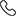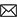Sales :9214233303 | 9214233343 / Tech. Support Desk :7231890000 (9AM ~ 6PM)# JEE Syllabus 2022

## IIT JEE Syllabus 2022

Find the Completes Syllabus of JEE Main & Advanced 2022 on Etoosindia. Get full Syllabus for Physics, Chemistry and Mathematics here.

To make students well-versed with Joint Entrance Exam (JEE), ETOOSINDIA brings you the complete IIT JEE Syllabus 2022 to start your preparation. JEE consists of JEE Main and JEE Advanced paper offering admissions to BE/B.Tech & B.Arch/B.Plan courses. The exam is a gateway to get into renowned IITs, NITs, IITs, CFTIs, other engineering & architecture colleges in India. The syllabus comprises of subjects Physics, Chemistry & Mathematics. Students need to cover the entire syllabus well-in time to crack this prestigious exam. So, begin you your preparation for JEE 2022 with ETOOSINDIA. The updated syllabus is mentioned below.

##### PHYSICS
S.No. Syllabus S.No. Syllabus
1 General: Units and dimensions 1 Mechanics
2 Thermal physics 2 Electricity and Magnetism
3 Electromagnetic Induction 3 Optics
4 Wave Nature of light 4 Modern Physics
5 Electronic Devices 5 Communication Systems
##### Chemistry
S.No. Physical Chemistry Inorganic Chemistry Organic Chemistry
1 General topics Classification of elements
and periodicity in properties
Concepts
2 Gaseous and liquid states Isolation/preparation and
properties of the following non-metals
Purification and Characterisation
of organic compounds
3 Energetics Hydrogen Some Basic Principles of Organic Chemistry
4 Chemical Equilibrium S- Block Elements Preparation, Properties and Reactions of Alkanes
5 Ionic Equilibrium P Block Elements
6 Redox and Electrochemistry D and F Block Elements Hydrocarbons
7 Chemical Kinetics Coordination Compounds Organic compounds containing halogens
8 Solid State Atoms and Nuclei Preparation, properties and reactions of alkenes and alkynes
9 Solutions Environmental Chemistry Reactions of Benzene
10 Surface Chemistry Preparation and properties of compounds:
Oxides, peroxides, hydroxides
Alcohols
11 Nuclear Chemistry Transition Elements Phenols
12 Ores and Minerals Ethers
13 Extractive Metallurgy Aldehydes and Ketones
14 Principles of qualitative analysis Amines and Diazonium Salt
15 Carbohydrates
15 Characteristic Reactions
16 Chemistry in Everyday life
17 Amino Ccids and Peptides
18 Properties and uses of
some important polymers
19 Practical Organic Chemistry
##### Mathematics
S.No. Syllabus S.No. Syllabus
2 Logarithms 2 Sequence & Series
3 Mathematical Induction 3 Permutations and Combinations, Binomial Theorem
4 Matrices 4 Statistics
5 Trigonometry 5 Analytical Geometry
6 Differential Calculus 6 Integral calculus
7 Vectors 7 Mathematical Reasoning
##### Mathematics

Algebra Algebra of complex numbers, addition, multiplication, conjugation, polar representation, properties of modulus and principal argument, triangle inequality, cube roots of unity, geometric interpretations.

Quadratic equations with real coefficients, relations between roots and coefficients, formation of quadratic equations with given roots, symmetric functions of roots.
Arithmetic, geometric and harmonic progressions, arithmetic, geometric and harmonic means, sums of finite arithmetic and geometric progressions infinite geometric series, sums of squares and cubes of the first n natural numbers

Logarithms and their properties Sequence & Series Arithmetic & geometric progression insertion of arithmetic, geometric means between two given numbers, Relation between A.M & G.M . Sum upto n terms of special series: Sn, Sn2, Sn3 . Arithmetic ? geometric progression!

Mathematical Induction Principle of Mathematics Induction & its simple applications.

Permutations and combinations, Binomial theorem for a positive integral index, properties of binomial coefficients.

Matrices as a rectangular array of real numbers, equality of matrices, addition, multiplication by a scalar and product of matrices, transpose of a matrix, determinant of a square matrix of order up to three, inverse of a square matrix of order up to three, properties of these matrix operations diagonal, symmetric and skew-symmetric matrices and their properties, solutions of simultaneous linear equations in two or three variables. Addition and multiplication rules of probability, conditional probability, Bayes Theorem, independence of events, computation of probability of events using permutations and combinations.

Statistics Measure of Dispersion. Calculation of mean, median, mode of grouped & ungrouped data calculation of standard deviation, variance and mean deviation for grouped & ungrouped data.

Trigonometry : Trigonometric identities & functions, their periodicity and graphs, addition and subtraction formulae, formulae involving multiple and sub-multiple angles, general solution of trigonometric equations. Relations between sides and angles of a triangle, sine rule, cosine rule, half-angle formula and the area of a triangle, inverse trigonometric functions (principal value only).Analytical Geometry Two dimensions: Cartesian coordinates, distance between two points, section formulae, shift of origin. Equation of a straight line in various forms, angle between two lines, distance of a point from a line. Lines through the point of intersection of two given lines, equation of the bisector of the angle between two lines, concurrency of lines, centroid, orthocentre, incentre and circumcentre of a triangle

Equation of a circle in various forms, equations of tangent, normal and chord. Parametric equations of a circle, intersection of a circle with a straight line or a circle, equation of a circle through the points of intersection of two circles and those of a circle and a straight line. Equations of a parabola, ellipse and hyperbola in standard form, their foci, directrices and eccentricity, parametric equations, equations of tangent and normal. Locus Problems.

Three dimensions: Direction cosines and direction ratios, equation of a straight line in space, equation of a plane, distance of a point from a plane.

Differential Calculus Real valued functions of a real variable, into, onto and one-to-one functions, sum, difference, product and quotient of two functions, composite functions, absolute value, polynomial, rational, trigonometric, exponential and logarithmic functions.

Integral Calculus Integration as the inverse process of differentiation, indefinite integrals of standard functions, definite integrals and their properties, application of the Fundamental Theorem of Integral Calculus.

Vectors Addition of vectors, scalar multiplication, scalar products, dot and cross products, scalar triple products and their geometrical interpretations.